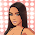## Wednesday, January 30, 2013

### Maximum Likelihood Estimation

Maximum Likelihood Estimation is widely applicable method for estimating the parameters of a probabilistic model.

Developed by R.A.Fisher in the 1920s the principle behind it is that the ideal parameter settings of a model are the ones that make the observed data most likely.

It is applicable in any situation in which the model can be specified such that the probability of the desired variable y can be expressed as a parameterised function over the vector of observed variables (X).

P(y|X) = f(X,φ)

The parameters φ of the function f(X, φ) are what we want to estimate.

The model is designed to be a function in which the parameters are set and we get back a probability value for a given x. However, we need a process to determine these model parameters. The Likelihood function is defined to be equal to this function, but operating as a function over the parameter space of φ.

L(φ | y,X )= P(y|X,φ)

It is important to recognise that Likelihood is not the probability of the parameters, it is just equal to the probability of y given the parameters. As such it is not a probability distribution over φ.

If we have N observations in our data set, and we let D represent all N of these observations of X and y, then we can express the Likelihood function for this entire data set D as :

L(φ | D ) = Ni=1 P(yi|Xi,φ )

Maximum Likelihood is then simply defined as Argmax φ over this function. Finding the value of φ that maximises this function can be done a number of ways.

To find an analytical solution to the Likelihood equation we find the partial derivative of the function with respect to each of the paramters. We then solve this series of equations for the parameter values such the the partial derivatives are equal to zero. This gives us a position that is either a max or min. We then find the second partial derivative with respect to each parameter and make sure it is negative at the points found in the first step. This will give us an analytical peak on the Likelihood surface.

The reality of maximising the Likelihood by searching the parameter space depends a great deal on the problem. Numerous tricks occur to simplify the problem. The natural logarithm of the Likelihood function is often taken because they are monotonically related, so the MLE can be obtained by maximising the log of the Likelihood. In addition, taking the log turns the product into a Sum and can improves the chance of finding an analytical solution, and improve the computational tractability of finding a numerical solution.

In the next post I will summarise the use of the Expectation Maximisation algorithm for situations in which the Likelihood function cannot be solved analytically.

1.2.